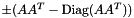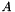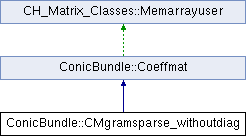ConicBundle
ConicBundle::CMgramsparse_withoutdiag Class Reference

implements a Gram matrixwith zero diagonal as Coeffmat for a sparse rectangular CH_Matrix_Classes::Sparsemat(for use with MatrixSDPfunction, see implemention of a PSCOracle (PSCAffineFunction)). More...

#include <CMgramsparse_withoutdiag.hxx>

Inheritance diagram for ConicBundle::CMgramsparse_withoutdiag:## Public Member Functions

CMgramsparse_withoutdiag (const CH_Matrix_Classes::Sparsemat &Ain, bool pos=true, CoeffmatInfo *cip=0)
copy Ain, the flag for positive/negative and store the user information

virtual Coeffmatclone () const
makes an explicit copy of itself and returns a pointer to it

virtual CH_Matrix_Classes::Integer dim () const
returns the order of the represented symmetric matrix

virtual CH_Matrix_Classes::Real operator() (CH_Matrix_Classes::Integer i, CH_Matrix_Classes::Integer j) const
returns the value of the matrix element (i,j)

virtual void make_symmatrix (CH_Matrix_Classes::Symmatrix &S) const
returns a dense symmetric constraint matrix

virtual CH_Matrix_Classes::Real norm (void) const
returns the Frobenius norm of the matrix

virtual Coeffmatsubspace (const CH_Matrix_Classes::Matrix &P) const
delivers a new object on the heap corresponding to the matrix P^T(*this)P, the caller is responsible for deleting the object

virtual void multiply (CH_Matrix_Classes::Real d)
multiply constraint permanentely by d; this is to allow scaling or sign changes in the constraints

virtual CH_Matrix_Classes::Real ip (const CH_Matrix_Classes::Symmatrix &S) const
returns ip(*this,S)=trace(*this*S), the trace inner product

virtual CH_Matrix_Classes::Real gramip (const CH_Matrix_Classes::Matrix &P) const
returns ip(*this,PP^T)=trace P^T(*this)P

virtual CH_Matrix_Classes::Real gramip (const CH_Matrix_Classes::Matrix &P, CH_Matrix_Classes::Integer start_row, const CH_Matrix_Classes::Matrix *Lam=0) const
returns ip(*this,QQ^T)=trace Q^T(*this)Q for Q=P.rows(start_row,start_row+dim-1)

virtual void addmeto (CH_Matrix_Classes::Symmatrix &S, CH_Matrix_Classes::Real d=1.) const
computes S+=d*(*this);

virtual void addprodto (CH_Matrix_Classes::Matrix &B, const CH_Matrix_Classes::Matrix &C, CH_Matrix_Classes::Real d=1.) const
computes B+=d*(*this)*C

virtual void addprodto (CH_Matrix_Classes::Matrix &B, const CH_Matrix_Classes::Sparsemat &C, CH_Matrix_Classes::Real d=1.) const
computes B+=d*(*this)*C

virtual void left_right_prod (const CH_Matrix_Classes::Matrix &P, const CH_Matrix_Classes::Matrix &Q, CH_Matrix_Classes::Matrix &R) const
computes R=P^T*(*this)*Q

virtual CH_Matrix_Classes::Integer prodvec_flops () const
returns an estimate of number of flops to compute addprodto for a vector

virtual int dense () const
returns 1 if its structure is as bad as its dense symmetric representation, otherwise 0

virtual int sparse () const
returns 0 if not sparse, otherwise 1

virtual int sparse (CH_Matrix_Classes::Indexmatrix &, CH_Matrix_Classes::Indexmatrix &, CH_Matrix_Classes::Matrix &, CH_Matrix_Classes::Real) const
returns 0 if not sparse. If it is sparse it returns 1 and the nonzero structure in I,J and v, where v is multiplied by d. Only the upper triangle (including diagonal) is delivered

virtual int support_in (const CH_Matrix_Classes::Sparsesym &) const
returns 0 if the support of the costraint matrix is not contained in the support of the sparse symmetric matrix S, 1 if it is contained.

virtual CH_Matrix_Classes::Real ip (const CH_Matrix_Classes::Sparsesym &S) const
returns the inner product of the constraint matrix with S

virtual void project (CH_Matrix_Classes::Symmatrix &S, const CH_Matrix_Classes::Matrix &P) const
computes S=P^T*(*this)*P

virtual void add_projection (CH_Matrix_Classes::Symmatrix &S, const CH_Matrix_Classes::Matrix &P, CH_Matrix_Classes::Real alpha=1., CH_Matrix_Classes::Integer start_row=0) const
computes S+=Q^T(*this)Q for Q=P.rows(start_row,start_row+dim-1)

virtual const CH_Matrix_Classes::Matrixpostgenmult (const CH_Matrix_Classes::Matrix &B, CH_Matrix_Classes::Matrix &C, CH_Matrix_Classes::Real alpha=1., CH_Matrix_Classes::Real beta=0., int btrans=0) const
computes C= alpha*(*this)*B^(T if btrans) + beta*C, C is also returned

virtual const CH_Matrix_Classes::Matrixpregenmult (const CH_Matrix_Classes::Matrix &B, CH_Matrix_Classes::Matrix &C, CH_Matrix_Classes::Real alpha=1., CH_Matrix_Classes::Real beta=0., int btrans=0) const
computes C= alpha*B^(T if btrans)*(*this) + beta*C, C is also returned

virtual int equal (const Coeffmat *p, double tol=1e-6) const
returns 1, if p is the same derived class and entries differ by less than tol, otherwise zero

virtual std::ostream & display (std::ostream &o) const
display constraint information

virtual std::ostream & out (std::ostream &o) const
put entire contents onto ostream with the class type in the beginning so that the derived class can be recognized by in().

virtual std::istream & in (std::istream &i)
counterpart to out(), does not read the class type, though. This is assumed to have been read in order to generate the correct class

CMgramsparse_withoutdiag (std::istream &is, CoeffmatInfo *cip=0)
constructor with istream and possibly additional user information

const CH_Matrix_Classes::Sparsematget_A () const
returns the const reference to the internal matrix A forming the Gram matrix

bool get_positive () const
returns the flag on whether the Gram matrix is used in positive or negative formPublic Member Functions inherited from ConicBundle::Coeffmat
Coeffmat (bool del_by_CoeffmatPointer=true)
default constructor; set del_by_CoeffmatPointer==true if a CoeffmatPointer reducing the use_cnt to zero should delete this

void set_deletion_by_CoeffmatPointer (bool dbMP)
if set to true any CoeffmatPointer that reduces use_cnt to zero will delete this object

virtual Coeffmattype get_type () const
returns its Coeffmattype

virtual CoeffmatInfoget_info ()
returns the user information

virtual const CoeffmatInfoget_info () const
returns the user information in const form

virtual void set_info (CoeffmatInfo *cip)
deletes the old and sets new user information

## Private Attributes

CH_Matrix_Classes::Sparsemat A
the Coeffmat acts like A*A^T-Diag(A*A^T) or its negative

CH_Matrix_Classes::Sparsesym di
this is the precomputed diagonal di=diag(A*A^T)

bool positive
if true use A*A^T-Diag, if false use -A*A^T+DiagProtected Member Functions inherited from CH_Matrix_Classes::Memarrayuser
Memarrayuser ()
if memarray is NULL, then a new Memarray is generated. In any case the number of users of the Memarray is incremented

virtual ~Memarrayuser ()
the number of users is decremented and the Memarray memory manager is destructed, if the number is zero.Protected Attributes inherited from ConicBundle::Coeffmat
Coeffmattype CM_type
in order to enable type identification

CoeffmatInfoinfop
allows the user to specify and output additional informationStatic Protected Attributes inherited from CH_Matrix_Classes::Memarrayuser
static Memarraymemarray
pointer to common memory manager for all Memarrayusers, instantiated in memarray.cxx

## Detailed Description

implements a Gram matrixwith zero diagonal as Coeffmat for a sparse rectangular CH_Matrix_Classes::Sparsemat(for use with MatrixSDPfunction, see implemention of a PSCOracle (PSCAffineFunction)).

As for CMgramsparse there is an extra flag for using the positive or the negative version of the matrix.

The documentation for this class was generated from the following file: